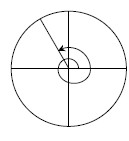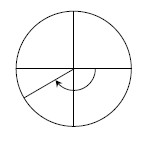### Home > PC > Chapter 3 > Lesson 3.3.1 > Problem3-110

3-110.

Below are two of the special angles that are used in the unit circle. Identify the radian measure for each angle shown. Remember counter-clockwise angles are positive and clockwise angles are negative.

1.1.Divide each circle into equal sectors and count them.
Remember that half of a circle is equivalent to $π$ radians.

Review problems 3-12 and 3-94.